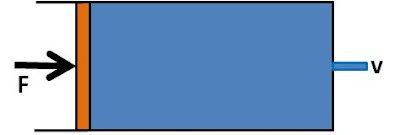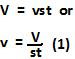## Saturday, May 23, 2009

### Irodov Problem 1.322The force F acting upon the fluid to push it out is constant and hence the pressure exerted on the fluid is also constant. This in turn means that the velocity with which the water jets out of the orifice will also be constant (due to Bernoulli's equation, since pressure head does not change). Let this velocity be v. We also know that the surface area of the orifice is s, so the rate at which water is flowing out of the orifice is given by vs (volume of flow per unit time). Since it took a t seconds for the water to flow out completely and the total volume of water was V, we have,The entire work done by the force is essentially converted into the kinetic energy of the water jetting out of the cylinder. Since the mass of the water is, the total kinetic energy imparted by to the entire volume of water and hence the work done given by,